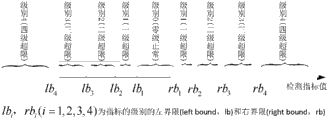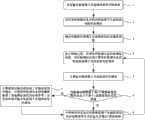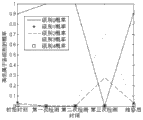# CN102518012A - 融合多种设备检测数据进行轨道检测的方法 - Google Patents

## Info

Publication number
CN102518012A
CN102518012A CN2011103700219A CN201110370021A CN102518012A CN 102518012 A CN102518012 A CN 102518012A CN 2011103700219 A CN2011103700219 A CN 2011103700219A CN 201110370021 A CN201110370021 A CN 201110370021A CN 102518012 A CN102518012 A CN 102518012A
Authority
CN
China
Prior art keywords
level
transfinites
detection
probability
standard
Prior art date
Legal status (The legal status is an assumption and is not a legal conclusion. Google has not performed a legal analysis and makes no representation as to the accuracy of the status listed.)
Granted
Application number
CN2011103700219A
Other languages
English (en)
Other versions
CN102518012B (zh
Inventor

Current Assignee (The listed assignees may be inaccurate. Google has not performed a legal analysis and makes no representation or warranty as to the accuracy of the list.)
Beijing Jiaotong University
Original Assignee
Beijing Jiaotong University
Priority date (The priority date is an assumption and is not a legal conclusion. Google has not performed a legal analysis and makes no representation as to the accuracy of the date listed.)
Filing date
Publication date
Application filed by Beijing Jiaotong University filed Critical Beijing Jiaotong University
Priority to CN201110370021.9A priority Critical patent/CN102518012B/zh
Publication of CN102518012A publication Critical patent/CN102518012A/zh
Application granted granted Critical
Publication of CN102518012B publication Critical patent/CN102518012B/zh
Active legal-status Critical Current
Anticipated expiration legal-status Critical

## Images

•••## Description

${f}_{0}\left(x\right)=\left\{\begin{array}{c}\mathrm{exp}\left[\frac{-{\left(x-\frac{{\mathrm{lb}}_{1}+{\mathrm{rb}}_{1}}{2}\right)}^{2}}{{\left(\frac{{\mathrm{lb}}_{1}-{\mathrm{rb}}_{1}}{2}\right)}^{2}}×\mathrm{ln}2\right],{\mathrm{lb}}_{1} 其中，lb1和rb1为检测指标值属于正常标准级的左界限和右界限。

${f}_{i}\left(x\right)=\left\{\begin{array}{c}\mathrm{exp}\left[\frac{-{\left(x-\frac{{\mathrm{lb}}_{i}+{\mathrm{lb}}_{i+1}}{2}\right)}^{2}}{{\left(\frac{{\mathrm{lb}}_{i}-{\mathrm{lb}}_{i+1}}{2}\right)}^{2}}×\mathrm{ln}2\right],{\mathrm{lb}}_{i+1}≤x≤{\mathrm{lb}}_{i}\\ {d}_{i}\mathrm{exp}\left[\frac{-{\left(x-\frac{{\mathrm{rb}}_{i}+{\mathrm{rb}}_{i+1}}{2}\right)}^{2}}{{\left(\frac{{\mathrm{rb}}_{i}-{\mathrm{rb}}_{i+1}}{2}\right)}^{2}}×\mathrm{ln}2\right],{\mathrm{rb}}_{i}≤x≤{\mathrm{rb}}_{i+1}\\ \frac{1}{1+\mathrm{exp}\left(-x+{\mathrm{lb}}_{i+1}\right)},x≤{\mathrm{lb}}_{i+1}\\ \frac{1}{1+\mathrm{exp}\left(x-{\mathrm{lb}}_{i}\right)},{\mathrm{lb}}_{i}≤x≤\frac{{\mathrm{lb}}_{1}+{\mathrm{rb}}_{1}}{2}\\ \frac{1}{1+\mathrm{exp}\left(-x+{\mathrm{rb}}_{i}\right)},\frac{{\mathrm{lb}}_{1}+{\mathrm{rb}}_{1}}{2}

${f}_{4}\left(x\right)=\left\{\begin{array}{c}\frac{1}{1+\mathrm{exp}\left(x-{\mathrm{lb}}_{4}\right)},x≤\frac{{\mathrm{lb}}_{1}+{\mathrm{rb}}_{1}}{2}\\ \frac{1}{1+\mathrm{exp}\left(-x+{\mathrm{rb}}_{4}\right)},x>\frac{{\mathrm{lb}}_{1}+{\mathrm{rb}}_{1}}{2}\end{array}\right\$

${f}_{0}\left(x\right)=\left\{\begin{array}{c}{a}_{0}\mathrm{exp}\left[\frac{-{\left(x-{b}_{0}\right)}^{2}}{{{c}_{0}}^{2}}\right],{\mathrm{lb}}_{1}≤x≤{\mathrm{rb}}_{1}\\ \frac{1}{1+\mathrm{exp}\left(-x+{d}_{0}\right)},x≤{\mathrm{lb}}_{1}\\ \frac{1}{1+\mathrm{exp}\left(x-{e}_{0}\right)},x≥{\mathrm{rb}}_{1}\end{array}\right\$

f0(lb1)＝0.5，f0(rb1)＝0.5，则可以得到f0(x)为：
${f}_{0}\left(x\right)=\left\{\begin{array}{c}\mathrm{exp}\left[\frac{-{\left(x-\frac{{\mathrm{lb}}_{1}+{\mathrm{rb}}_{1}}{2}\right)}^{2}}{{\left(\frac{{\mathrm{lb}}_{1}-{\mathrm{rb}}_{1}}{2}\right)}^{2}}×\mathrm{ln}2\right],{\mathrm{lb}}_{1}

${f}_{i}\left(x\right)=\left\{\begin{array}{c}{a}_{i}\mathrm{exp}\left[\frac{-{\left(x-{b}_{i}\right)}^{2}}{{{c}_{i}}^{2}}\right],{\mathrm{lb}}_{i+1}≤x≤{\mathrm{lb}}_{i}\\ {d}_{i}\mathrm{exp}\left[\frac{-{\left(x-{e}_{i}\right)}^{2}}{{{f}_{i}}^{2}}\right],{\mathrm{rb}}_{i}≤x≤{\mathrm{rb}}_{i+1}\\ \frac{1}{1+\mathrm{exp}\left(-x+{g}_{i}\right)},x≤{\mathrm{lb}}_{i+1}\\ \frac{1}{1+\mathrm{exp}\left(x-{h}_{i}\right)},{\mathrm{lb}}_{i}≤x≤\frac{{\mathrm{lb}}_{1}+{\mathrm{rb}}_{1}}{2}\\ \frac{1}{1+\mathrm{exp}\left(-x+{j}_{i}\right)},\frac{{\mathrm{lb}}_{1}+{\mathrm{rb}}_{1}}{2}

${f}_{i}\left(x\right)=\left\{\begin{array}{c}\mathrm{exp}\left[\frac{-{\left(x-\frac{{\mathrm{lb}}_{i}+{\mathrm{lb}}_{i+1}}{2}\right)}^{2}}{{\left(\frac{{\mathrm{lb}}_{i}-{\mathrm{lb}}_{i+1}}{2}\right)}^{2}}·\mathrm{ln}2\right],{\mathrm{lb}}_{i+1}≤x≤{\mathrm{lb}}_{i}\\ {d}_{i}\mathrm{exp}\left[\frac{-{\left(x-\frac{{\mathrm{rb}}_{i}+{\mathrm{rb}}_{i+1}}{2}\right)}^{2}}{{\left(\frac{{\mathrm{rb}}_{i}-{\mathrm{rb}}_{i+1}}{2}\right)}^{2}}·\mathrm{ln}2\right],{\mathrm{rb}}_{i}≤x≤{\mathrm{rb}}_{i+1}\\ \frac{1}{1+\mathrm{exp}\left(-x+{\mathrm{lb}}_{i+1}\right)},x≤{\mathrm{lb}}_{i+1}\\ \frac{1}{1+\mathrm{exp}\left(x-{\mathrm{lb}}_{i}\right)},{\mathrm{lb}}_{i}≤x≤\frac{{\mathrm{lb}}_{1}+{\mathrm{rb}}_{1}}{2}\\ \frac{1}{1+\mathrm{exp}\left(-x+{\mathrm{rb}}_{i}\right)},\frac{{\mathrm{lb}}_{1}+{\mathrm{rb}}_{1}}{2}

${f}_{4}\left(x\right)=\left\{\begin{array}{c}\frac{1}{1+\mathrm{exp}\left(x+{a}_{1}^{′}\right)},x≤\frac{{\mathrm{lb}}_{1}+{\mathrm{rb}}_{1}}{2}\\ \frac{1}{1+\mathrm{exp}\left(-x+{a}_{2}^{′}\right)},x>\frac{{\mathrm{lb}}_{1}+{\mathrm{rb}}_{1}}{2}\end{array}\right\$

f4(lb4)＝0.5，f4(rb4)＝0.5

${f}_{4}\left(x\right)=\left\{\begin{array}{c}\frac{1}{1+\mathrm{exp}\left(x-{\mathrm{lb}}_{4}\right)},x≤\frac{{\mathrm{lb}}_{1}+{\mathrm{rb}}_{1}}{2}\\ \frac{1}{1+\mathrm{exp}\left(-x+{\mathrm{rb}}_{4}\right)},x>\frac{{\mathrm{lb}}_{1}+{\mathrm{rb}}_{1}}{2}\end{array}\right\$

lb1＝-8，rb1＝8，lb2＝-12，rb2＝12，lb3＝-20，rb3＝20，lb4＝-30，rb4＝30。

lb1＝-9，rb1＝9，lb2＝-14，rb2＝14，lb3＝-22，rb3＝22，lb4＝-34，rb4＝34。

lb1＝-6，rb1＝6，lb2＝-10，rb2＝10，lb3＝-19，rb3＝19，lb4＝-26，rb4＝26。

## Claims (4)

1.一种融合多种设备检测数据进行轨道检测的方法，其特征是所述方法包括：

2.根据权利要求1所述的方法，其特征是所述确定检测指标值属于各超限级别的隶属度函数fi(x)具体是，当超限级别为正常标准级时，检测指标值属于正常标准级的隶属度函数f0(x)为：
${f}_{0}\left(x\right)=\left\{\begin{array}{c}\mathrm{exp}\left[\frac{-{\left(x-\frac{{\mathrm{lb}}_{1}+{\mathrm{rb}}_{1}}{2}\right)}^{2}}{{\left(\frac{{\mathrm{lb}}_{1}-{\mathrm{rb}}_{1}}{2}\right)}^{2}}×\mathrm{ln}2\right],{\mathrm{lb}}_{1} 其中，lb1和rb1为检测指标值属于正常标准级的左界限和右界限。
3.根据权利要求1所述的方法，其特征是所述确定检测指标值属于各超限级别的隶属度函数fi(x)具体是，当超限级别为保养标准级、舒适度标准级或者临时补修标准级时，检测指标值属于保养标准级、舒适度标准级或者临时补修标准级的隶属度函数fi(x)为：
${f}_{i}\left(x\right)=\left\{\begin{array}{c}\mathrm{exp}\left[\frac{-{\left(x-\frac{{\mathrm{lb}}_{i}+{\mathrm{lb}}_{i+1}}{2}\right)}^{2}}{{\left(\frac{{\mathrm{lb}}_{i}-{\mathrm{lb}}_{i+1}}{2}\right)}^{2}}×\mathrm{ln}2\right],{\mathrm{lb}}_{i+1}≤x≤{\mathrm{lb}}_{i}\\ {d}_{i}\mathrm{exp}\left[\frac{-{\left(x-\frac{{\mathrm{rb}}_{i}+{\mathrm{rb}}_{i+1}}{2}\right)}^{2}}{{\left(\frac{{\mathrm{rb}}_{i}-{\mathrm{rb}}_{i+1}}{2}\right)}^{2}}×\mathrm{ln}2\right],{\mathrm{rb}}_{i}≤x≤{\mathrm{rb}}_{i+1}\\ \frac{1}{1+\mathrm{exp}\left(-x+{\mathrm{lb}}_{i+1}\right)},x≤{\mathrm{lb}}_{i+1}\\ \frac{1}{1+\mathrm{exp}\left(x-{\mathrm{lb}}_{i}\right)},{\mathrm{lb}}_{i}≤x≤\frac{{\mathrm{lb}}_{1}+{\mathrm{rb}}_{1}}{2}\\ \frac{1}{1+\mathrm{exp}\left(-x+{\mathrm{rb}}_{i}\right)},\frac{{\mathrm{lb}}_{1}+{\mathrm{rb}}_{1}}{2}

4.根据权利要求1所述的方法，其特征是所述确定检测指标值属于各超限级别的隶属度函数fi(x)具体是，当超限级别为限速标准级时，检测指标值属于限速标准级的隶属度函数f4(x)为：
${f}_{4}\left(x\right)=\left\{\begin{array}{c}\frac{1}{1+\mathrm{exp}\left(x-{\mathrm{lb}}_{4}\right)},x≤\frac{{\mathrm{lb}}_{1}+{\mathrm{rb}}_{1}}{2}\\ \frac{1}{1+\mathrm{exp}\left(-x+{\mathrm{rb}}_{4}\right)},x>\frac{{\mathrm{lb}}_{1}+{\mathrm{rb}}_{1}}{2}\end{array}\right\$

CN201110370021.9A 2011-11-18 2011-11-18 融合多种设备检测数据进行轨道检测的方法 Active CN102518012B (zh)

## Priority Applications (1)

Application Number Priority Date Filing Date Title
CN201110370021.9A CN102518012B (zh) 2011-11-18 2011-11-18 融合多种设备检测数据进行轨道检测的方法

## Applications Claiming Priority (1)

Application Number Priority Date Filing Date Title
CN201110370021.9A CN102518012B (zh) 2011-11-18 2011-11-18 融合多种设备检测数据进行轨道检测的方法

## Publications (2)

Publication Number Publication Date
CN102518012A true CN102518012A (zh) 2012-06-27
CN102518012B CN102518012B (zh) 2014-05-28

# Family

## Family Applications (1)

Application Number Title Priority Date Filing Date
CN201110370021.9A Active CN102518012B (zh) 2011-11-18 2011-11-18 融合多种设备检测数据进行轨道检测的方法

## Country Status (1)

CN (1) CN102518012B (zh)

## Cited By (2)

* Cited by examiner, † Cited by third party
Publication number Priority date Publication date Assignee Title
CN103434537A (zh) * 2013-08-27 2013-12-11 中国神华能源股份有限公司 铁路线路预警方法和系统
CN113032907A (zh) * 2021-03-26 2021-06-25 北京交通大学 基于波形相关关系的晃车病害数据偏差纠正方法及系统

## Citations (5)

* Cited by examiner, † Cited by third party
Publication number Priority date Publication date Assignee Title
US5867404A (en) * 1996-04-01 1999-02-02 Cairo Systems, Inc. Method and apparatus for monitoring railway defects
CN1369595A (zh) * 2002-03-15 2002-09-18 西南交通大学 轨道状态检测装置
JP2005350895A (ja) * 2004-06-08 2005-12-22 Hashizume Kiko Kk 軌道補修方法および軌道補修装置
CN101142462A (zh) * 2004-06-30 2008-03-12 乔治敦铁轨设备公司 用于检查铁路轨道的系统和方法
CN201835196U (zh) * 2010-09-28 2011-05-18 中国铁道科学研究院基础设施检测研究所 轨道几何检测系统

## Patent Citations (5)

* Cited by examiner, † Cited by third party
Publication number Priority date Publication date Assignee Title
US5867404A (en) * 1996-04-01 1999-02-02 Cairo Systems, Inc. Method and apparatus for monitoring railway defects
CN1369595A (zh) * 2002-03-15 2002-09-18 西南交通大学 轨道状态检测装置
JP2005350895A (ja) * 2004-06-08 2005-12-22 Hashizume Kiko Kk 軌道補修方法および軌道補修装置
CN101142462A (zh) * 2004-06-30 2008-03-12 乔治敦铁轨设备公司 用于检查铁路轨道的系统和方法
CN201835196U (zh) * 2010-09-28 2011-05-18 中国铁道科学研究院基础设施检测研究所 轨道几何检测系统

## Non-Patent Citations (2)

* Cited by examiner, † Cited by third party
Title

## Cited By (3)

* Cited by examiner, † Cited by third party
Publication number Priority date Publication date Assignee Title
CN103434537A (zh) * 2013-08-27 2013-12-11 中国神华能源股份有限公司 铁路线路预警方法和系统
CN113032907A (zh) * 2021-03-26 2021-06-25 北京交通大学 基于波形相关关系的晃车病害数据偏差纠正方法及系统
CN113032907B (zh) * 2021-03-26 2023-07-25 北京交通大学 基于波形相关关系的晃车病害数据偏差纠正方法及系统

## Also Published As

Publication number Publication date
CN102518012B (zh) 2014-05-28

## Similar Documents

Publication Publication Date Title
CN103852269B (zh) 高速列车运行动力学参数检测方法
CN103674578B (zh) 高速列车运行动力学性能状态的检测方法
CN104239694A (zh) 一种城轨列车转向架的故障预测与视情维修方法
CN105303832B (zh) 基于微波车辆检测器的高架桥路段交通拥堵指数计算方法
CN103196681B (zh) 基于转向架加速度的列车运行舒适度预测方法
CN108698622A (zh) 轨道状态评价方法、装置以及程序
CN104309435B (zh) 一种路面不平度在线辨识方法
CN104833534A (zh) 一种基于多源信息融合的列车走行系故障诊断装置及方法
CN103471865A (zh) 基于线性判别法的列车悬挂系统故障分离方法
CN105973457A (zh) 动车组车载平稳性监控装置及方法
CN103207097B (zh) 轨道刚度动态检测方法
US10379008B2 (en) Railway vehicle condition monitoring apparatus
CN104833535A (zh) 一种铁道车辆车轮踏面擦伤的检测方法
Sun et al. A signal analysis based hunting instability detection methodology for high-speed railway vehicles
CN108254179A (zh) 一种基于meemd排列熵的高速列车轮对轴承故障诊断方法
CN105929025A (zh) 一种基于时间与空间连续的车轮踏面与轨道故障检测方法
CN107403139A (zh) 一种城轨列车车轮扁疤故障检测方法
CN114169422A (zh) 基于车辆振噪联合测试的地铁钢轨波磨识别方法及系统
CN105740606A (zh) 一种基于可靠性gert模型的高速列车可靠性分析方法
CN107423692A (zh) 一种基于小波包能量熵的钢轨波磨故障检测方法
CN107609317A (zh) 一种钢桥疲劳评估荷载建模及疲劳评估方法
Han et al. A new binary wheel wear prediction model based on statistical method and the demonstration
CN104864949A (zh) 一种车辆动态称重方法及装置
CN102360454A (zh) 基于narx神经网络的轮轨力预测方法
CN105501250B (zh) 基于车载检测装置的列车轮对踏面擦伤故障诊断方法

## Legal Events

Date Code Title Description
C06 Publication
PB01 Publication
C10 Entry into substantive examination
SE01 Entry into force of request for substantive examination
C14 Grant of patent or utility model
GR01 Patent grant